# Congruent Triangles

Triangles are congruent when they have
exactly the same three sides and exactly the same three angles.

## What is "Congruent" ... ?

It means that one shape can become another using Turns, Flips and/or Slides:

Rotation## Congruent Triangles

When two triangles are congruent they will have exactly the same three sides and exactly the same three angles.

The equal sides and angles may not be in the same position (if there is a turn or a flip), but they are there.

### Same Sides is Enough

When the sides are the same the triangles are congruent.

For example: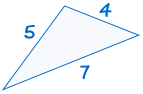is congruent to: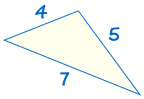and also to: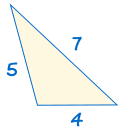because they all have exactly the same sides.

But:is NOT congruent to: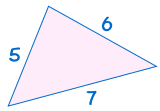because the two triangles do not have exactly the same sides.

### Same Angles?

Does this also work with angles? Not always!

Two triangles with the same angles might be congruent: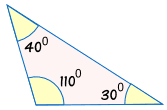is congruent to: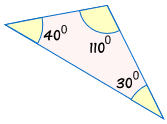only because they are the same size

But they might NOT be congruent because of different sizes:is NOT congruent to: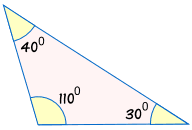all angles match, but one triangle is larger than the other!

So just having the same angles is no guarantee they are congruent.

### Other Combinations

There are other combinations of sides and angles that can work ...
... read more at How To Find if Triangles are Congruent

## Marking

When two triangles are congruent we often mark corresponding sides and angles like this: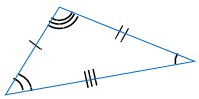is congruent to: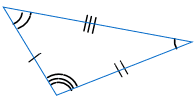The sides marked with one line are equal in length. Similarly for the sides marked with two lines. Also for the sides marked with three lines.

The angles marked with one arc are equal in size. Similarly for the angles marked with two arcs. Also for the angles marked with three arcs.

734, 735, 5026, 5027, 1524, 1525, 7492, 7493, 7494, 7495